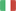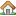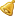EnglishItalianoVisitatore
Torna allaHomepage...

###Generali:

• Dipartimento: Ingegneria
• Tipologia: Corso Di Laurea Dm.270/04
• Corso di Laurea: Ingegneria Informatica
• Settore Ministeriale: MAT/05
• Codice di verbalizzazione: 8037589
• Metodi di insegnamento: Laboratorio
• Metodi di valutazione: Scritto E Orale
• Prerequisiti: High-school mathematics. Elementary algebra, geometry, trigonometry, algebraic equations and disequations of the first and second degree. Scompositions of elementary polynomials in isirreducible factors.
• Obiettivi: At the end of the course the students are supposed to be able to handle the mathematical tecniques learnt during the course. In particular the student are supposed to 1) compute the limit of a real funcion on one variable 2) to analyze the grafh on a real function of one variable 3) compute the derivatives and integrals a real function of one vriable 4) be able to study the bahavior of a real function of one variable in the neighborhood of a point and in particular how to use the Taylor expansion both with Peano and Lagrange "Rest" 5) be able to compute the 6) numerical series 7) improper integrals of real function of one real variable 8) limit of real functions of more than one variable 9) compute the partial derivatives 10) be able to establish the presence of the tangent plane wich is equivalent to the differentiability of the function.
• Ricevimento: Su appuntamento da fissarsi tramite posta elettronica

###Didattica:

• A.A.: 2018/2019
• Canale: UNICO
• Crediti (CFU): 12
• Obbligo di Frequenza: No